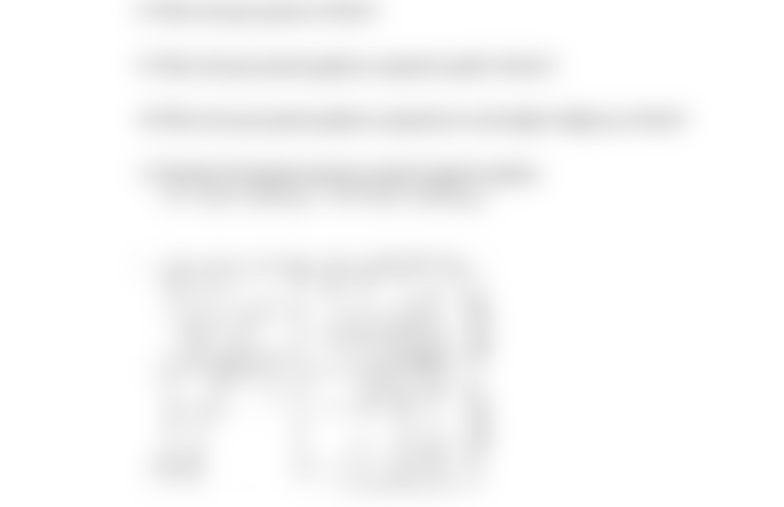Study Guides (390,000)
US (220,000)
LSU (10,000)
PETE (60)
All (3)
Final

# 4045class4 Eq Depth PPExam

Department
Petroleum Engineering
Course Code
PETE 4045
Professor
All
Study Guide
Final

This preview shows half of the first page. to view the full 1 pages of the document.Name: ______________________________
1
PETROLEUM ENGINEERING 4045 DRILLING ENGINEERING
IN-CLASS ASSIGNMENT # 4 FALL 2012
Pore Pressure Interpretation Using Equivalent Depth Method
1) What is the depth at “Point b?”
2) What is the “equivalent depth,” i.e. the depth in the normally pressured section with
the same porosity?
3) What is the formation pressure at the equivalent depth?
4) What is the overburden stress at the equivalent depth? Use overburden grad = 1 psi/ft
5) What is the effective stress at the equivalent depth?
6) What is the effective stress at Point b?
7) What is the overburden stress at Point b?
8) What is the pore pressure at Point b?
9) What is the pore pressure gradient, expressed in psi/ft, at Point b?
10) What is the pore pressure gradient, expressed as a mud weight in lb/gal eq, at Point b?
11) Calculate the formation pressure at point b using the equation:
))(/535.0())(/1( TVDnTVDf DftpsiDftpsiP